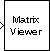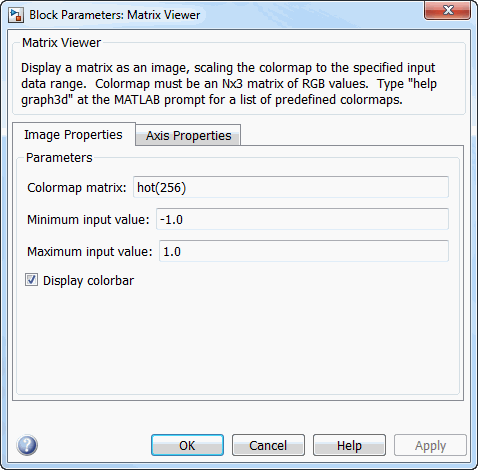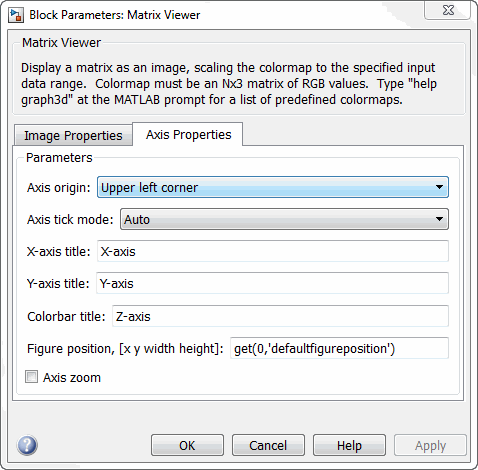Documentation

Matrix Viewer

Display matrices as color images

Library

Sinks

dspsnks4Description

The Matrix Viewer block displays an M-by-N matrix input by mapping the matrix element values to a specified range of colors. The display is updated as each new input is received. This block treats an unoriented length M vector input as an M-by-1 matrix.

You can use the Matrix Viewer block in models running in Normal or Accelerator simulation modes. The software does not support this block in models running in Rapid Accelerator or External mode. For more information about these modes, see How Acceleration Modes Work (Simulink) in the Simulink® User's Guide.

Image Properties

Select the Image Properties tab to show the image property parameters, which control the colormap and display.

You specify the mapping of matrix element values to colors in the Colormap matrix, Minimum input value, and Maximum input value parameters. For a colormap with L colors, the colormap matrix has dimension L-by-3, with one row for each color and one column for each element of the RGB triple that defines the color. Examples of RGB triples are

[ 1   0   0 ]   (red)
[ 0   0   1 ]   (blue)
[0.8 0.8 0.8]   (light gray)

See the ColorSpec property in the MATLAB® documentation for complete information about defining RGB triples.

MATLAB provides a number of functions for generating predefined colormaps, such as hot, cool, bone, and autumn. Each of these functions accepts the colormap size as an argument, and can be used in the Colormap matrix parameter. For example, when you specify gray(128) for the Colormap matrix parameter, the matrix is displayed in 128 shades of gray. The color in the first row of the colormap matrix represents the value specified by the Minimum input value parameter, and the color in the last row represents the value specified by the Maximum input value parameter. Values between the minimum and maximum are quantized and mapped to the intermediate rows of the colormap matrix.

The documentation for the MATLAB colormap function provides complete information about specifying colormap matrices, and includes a complete list of the available colormap functions.

Axis Properties

Select the Axis Properties tab to show the axis property parameters, which control labeling and positioning.

The Axis origin parameter determines where the first element of the input matrix, U(1,1), is displayed. When you specify Upper left corner, the matrix is displayed in matrix orientation, with U(1,1) in the upper-left corner.

$\left[\begin{array}{cccc}{U}_{11}& {U}_{12}& {U}_{13}& {U}_{14}\\ {U}_{21}& {U}_{22}& {U}_{23}& {U}_{24}\\ {U}_{31}& {U}_{32}& {U}_{33}& {U}_{34}\\ {U}_{41}& {U}_{42}& {U}_{43}& {U}_{44}\end{array}\right]$

When you specify Lower left corner, the matrix is flipped vertically to image orientation, with U(1,1) in the lower-left corner.

$\left[\begin{array}{cccc}{U}_{41}& {U}_{42}& {U}_{43}& {U}_{44}\\ {U}_{31}& {U}_{32}& {U}_{33}& {U}_{34}\\ {U}_{21}& {U}_{22}& {U}_{23}& {U}_{24}\\ {U}_{11}& {U}_{12}& {U}_{13}& {U}_{14}\end{array}\right]$

Axis zoom, when selected, causes the image display to completely fill the figure window. Axis titles are not displayed. This option can also be selected from the pop-up menu that is displayed when you right-click in the figure window. When Axis zoom is cleared, the axis labels and titles are displayed in a gray border surrounding the image axes.

To customize the axis tick range, set Axis tick mode to User-defined and specify a two-element row vector, [Minvalue Maxvalue], in X-tick range and Y-tick range. The matrix data does not change even when the axes ticks change. For example, consider displaying a matrix on axes with ticks ranging from 0 to 10. When you set X-tick range and Y-tick range to [0 1], the range of ticks changes from [0 10] to [0 1].

Figure Window

The image title in the figure title bar is the same as the block title. The axis tick marks reflect the size of the input matrix; the x-axis is numbered from 1 to N (number of columns), and the y-axis is numbered from 1 to M (number of rows).

Right-click the image in the figure window to access the following menu items:

• Refresh erases all data on the scope display except for the most recent image.

• Autoscale recomputes the minimum and maximum input values to fit the range of values observed in a series of 10 consecutive inputs. The numerical limits selected by the autoscale feature are shown in the Minimum input value and Maximum input value parameters, where you can make further adjustments to them manually.

• Axis zoom, when selected, causes the image to completely fill the figure window. Axis titles are not displayed. When Axis zoom is cleared, the axis labels and titles are displayed in a gray border surrounding the scope axes. This option can also be set in the Axis Properties pane of the parameter dialog box.

• Colorbar, when selected, displays a bar with the specified colormap to the right of the image axes.

• Save Position automatically updates the Figure position parameter in the Axis Properties pane to reflect the figure window's current position and size on the screen. To make the scope window open at a particular location on the screen when the simulation runs, drag the window to the desired location, resize it, and select Save Position. The parameter dialog box must be closed when you select Save Position for the Figure position parameter to be updated.

Dialog Box

The Image Properties pane of the Matrix Viewer block appears as follows.Colormap matrix

A 3-column matrix defining the colormap as a set of RGB triples, or a call to a colormap-generating function such as hot or spring. See the ColorSpec property for complete information about defining RGB triples, and the MATLAB colormap function for a list of colormap-generating functions. Tunable (Simulink).

Minimum input value

The input value to be mapped to the color defined in the first row of the colormap matrix. Right-click in the figure window and select Autoscale from pop-up menu to set this parameter to the minimum value observed in a series of 10 consecutive matrix inputs. Tunable (Simulink).

Maximum input value

The input value to be mapped to the color defined in the last row of the colormap matrix. Right-click in the figure window and select Autoscale from the pop-up menu to set this parameter to the maximum value observed in a series of 10 consecutive matrix inputs. Tunable (Simulink).

Display colorbar

Select to display a bar with the selected colormap to the right of the image axes. Tunable (Simulink).

The Axis Properties pane of the Matrix Viewer block appears as follows.Axis origin

The position within the axes where the first element of the input matrix, U(1,1), is plotted; bottom left or top left. Tunable (Simulink).

Axis tick mode

The mode used to specify the axis tick range. When you select Auto, the x-axis tick range and y-axis tick range are chosen based on the input signal. When you select User-defined, the axis tick range is set based on the values you specify in X-tick range and Y-tick range. The default is Auto.

X-tick range

x-axis tick range, specified as a two-element row vector of real scalars, [Xmin Xmax]. This parameter applies only when you set Axis tick mode to User-defined. When you change X-tick range during simulation, the axis range on the Matrix Viewer block updates in real time. The default is [0 1].

Y-tick range

y-axis tick range, specified as a two-element row vector of real scalars, [Ymin Ymax]. This parameter applies only when you set Axis tick mode to User-defined. When you change Y-tick range during simulation, the axis range on the Matrix Viewer block updates in real time. The default is [0 1].

X-axis title

The text to be displayed below the x-axis. Tunable (Simulink).

Y-axis title

The text to be displayed to the left of the y-axis. Tunable (Simulink).

Colorbar title

The text to be displayed to the right of the color bar, when Display colorbar is currently selected. Tunable (Simulink).

Figure position, [x y width height]

A 4-element vector of the form [x y width height] specifying the position of the figure window, where (0,0) is the lower-left corner of the display. Tunable (Simulink).

Axis zoom

Resizes the image to fill the figure window. Tunable (Simulink).

Supported Data Types

PortSupported Data Types

Input

• Double-precision floating point

• Single-precision floating point

• Fixed point (signed and unsigned)

• Boolean

• 8-, 16-, and 32-bit signed integers

• 8-, 16-, and 32-bit unsigned integers